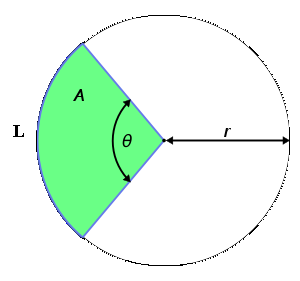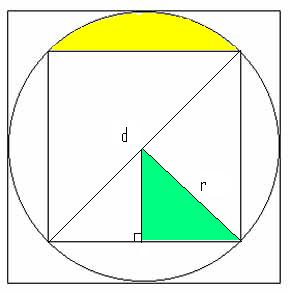# Geometry Series Part 3: Couple Common Circle Concepts:

by on November 20th, 2009

One of the key things to remember with circles is that once you know one piece of information, you know everything about the circle itself. Additional angles and lengths inside are not always so simple, but it is possible to convert circumferences to areas, to radii and diameters without intermediate steps. This will save you time in Data Sufficiency questions.

## Arc Lengths and Sector AreasArc Lengths (portions of perimeters) and Sectors (pie slices) seem more complicated than they really are. Both relate directly to the internal angle at the circle’s center, represented by θ in this diagram. Here are the equations:

Sector Area = A = θ/360 * πr²

Arc Length = L = θ/360 * 2πr

Literally, all we are doing is finding the fraction of the circle and applying it to either the area or circumference formula, respectively. Note there are only 2 variables. This is good to keep in mind for Data Sufficiency questions. It will be helpful to finding the solution to the following problem:In the above diagram, a square is inscribed in a circle, which is inscribed in another square. Which is larger, the green region or the yellow region?

(1) The area of the larger square is 64.
(2) The area of the green region is 4.

(Standard Data Sufficiency answer choices apply)

A(large square) = 64
s(large square) = 8
diameter = 8
r = 4

First, recognize that the figure is symmetrical. So while we may not explicitly be given an angle to find the sector area (not drawn) in which the yellow region resides, we do know its measure. The diagonals of a square intersect at a right angle, so we can deduce that the sector including the yellow region is 1/4 of the area of the circle. Since we know the radius…

A(large sector) = 1/4 * 16π = 4π

To find the yellow region itself, we must subtract the imaginary (not drawn) triangle from 4π. (Note that this imaginary triangle will be twice the green triangle.)

A(imaginary triangle) = 1/2 * r * r = 1/2 * 4 * 4 = 8

A(Yellow Region) = 4π – 8

The area of the green region can be found in two ways. Either we can see that it’s simply one-half of the 8 we just found, OR we can find both sides of the green triangle with the common 45-45-90 1:1:√2 formula. With a hypotenuse of 4, we derive 2√2 for each side, which yields an area of 4.

Which is greater,  4π – 8 or 4?

4π – 8. Sufficient. (We can save the calculations on the GMAT for DS questions, but it’s still good to go through it to practice for PS questions involving similar calculations.)

If we know the area of the green triangle equals 4, and that it is an isosceles right triangle, then we can set up a simple equation to find its sides, which can be denoted x:

1/2 * x² = 4
x² = 8
x = 2√2

If x = 2√2, then the hypotenuse (r) = 2√2 * √2 = 4. From here, we follow the same logic as we did for Statement 1, and determine Statement 2 is sufficient. The answer choice is D.

## One More ProblemA circular loop of wire is attached to the two straight wires of a dipole antenna at points X and Y. Point Z is the base of the antenna where the two straight wires meet. How far is point X from the center of the circular loop of wire?

(1) The perimeter of the circular loop of wire is 3 feet.
(2) The center of the circular loop of wire is 2 feet from point Z.

The key point to remember here is that all radii are equal. That is, the distance from the center of the circle (C, not drawn) to both X and Y is the same.

Statement 1: Before we mentioned any if you have any one piece of information about the circle, then you have it all. This is perfect example. If we know the circumference (perimeter) of the wire, we know the radius, regardless of the presence of Z in the diagram. Sufficient.

Statement 2: We can draw a line from Z to C, and mark that distance 2. Because both antenna are tangents to the circle, we know that angles CYZ and CXZ are both right angles, making 2 the hypotenuse. However, there is not additional information (angles or additional sides) that dictates the distance CX. You can visualize this lack of information; picture pinching point Z and dragging it “down” such that X and Y are still on the circle, but closer together. Since there is nothing restricting us from doing that, there is not enough information to accurately determine the length of either the two remaining sides of the right triangle. Insufficient. Answer Choice A.

Here are just a couple examples, but keep working hard. Givens in geometry provide a series of information, not just what’s stated. Make sure to keep that in mind for Data Sufficiency questions.

Good luck!

### Read other articles in this series:

•Hi,

Thank you for the article, it indeed has made me INGRAIN the functions of sectors and arcs. I have a question regarding the calculation of the imaginary triangle - shouldn't it be (8*4)/2. When you refer to imaginary triangle you refer to the one right below the yellow region? Sorry if this is a stupid question. I just didn't understand the chain of logic there.

All the best,
Jas

•You don't need any additional information to answer your first question. No matter what the radius, the ratio of the area of the yellow region to that of the green region is Pi -2 to 1, so the yellow region is larger. The statements are completely unnecessary.

•Yeah, I agree with Ian.

In the above diagram, a square is inscribed in a circle, which is inscribed in another square. Which is larger, the green region or the yellow region?

We can just answer the Question...!

Area of yellow region is : (π - 2) into the area of green region.

The answer choices stands no where.